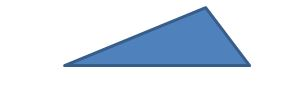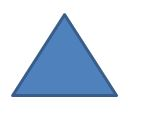Types of Triangles: Lesson for Kids

Instructor: Jennifer Zaur

Jennifer has taught at both the elementary and university level. She holds a master’s degree in curriculum and instruction.

In this lesson, you will learn about the three different types of triangles, which are scalene, isosceles, and equilateral. We will discuss how to identify each type of triangle.

What is a Triangle?

There are many different types of shapes, but one of the most common types of shapes is a triangle. A triangle is a shape that has three sides and three angles. Another fun fact about a triangle is the angles of a triangle always add up to 180 degrees.

Three Types of Triangles

So we know that a shape with three sides and angles is a triangle, but did you know that there is more than one type of triangle? The three main types of triangles are scalene, isosceles, and equilateral. In order to determine which type of triangle you have, you must look at the length of each of the sides of the triangle. Let's take a look at each one.

Scalene

The first and most common type of triangle is called a scalene triangle. A scalene triangle is a triangle that has no equal sides. In other words, the lengths of all of the sides of the triangle are different. Since none of the sides of a scalene triangle are equal, none of the angles are equal either.Isosceles

A second type of triangle is an isosceles triangle. An isosceles triangle is a triangle that has two equal sides. Since two sides are equal, one side is either long with two shorter sides or one side is short with two longer sides. On an isosceles triangle, two of the angles are also equal.Equilateral

A third type of triangle is an equilateral triangle. All three sides on an equilateral triangle are the same length or equal. Another way to say this is that all three sides of an equilateral triangle are congruent (the same). Along with all of the sides of an equilateral triangle being equal, all of the angles are equal as well.To unlock this lesson you must be a Study.com Member.

Register to view this lesson

Are you a student or a teacher?

See for yourself why 30 million people use Study.com

Become a Study.com member and start learning now.
Back
What teachers are saying about Study.com

Earning College Credit

Did you know… We have over 200 college courses that prepare you to earn credit by exam that is accepted by over 1,500 colleges and universities. You can test out of the first two years of college and save thousands off your degree. Anyone can earn credit-by-exam regardless of age or education level.